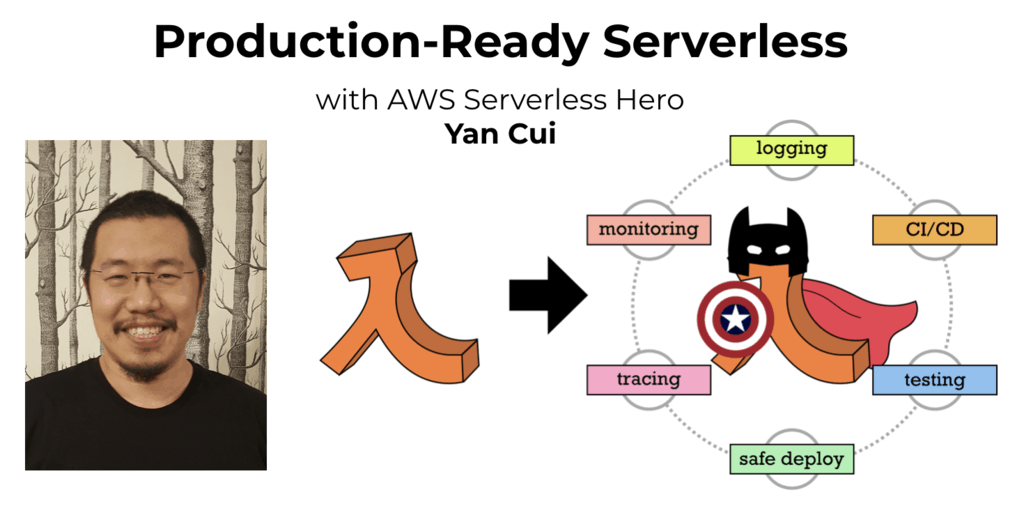# Project Euler – Problem 51 Solution

Check out my new course Learn you some Lambda best practice for great good! and learn the best practices for performance, cost, security, resilience, observability and scalability.

#### Problem

By replacing the 1st digit of *3, it turns out that six of the nine possible values: 13, 23, 43, 53, 73, and 83, are all prime.

By replacing the 3rd and 4th digits of 56**3 with the same digit, this 5-digit number is the first example having seven primes among the ten generated numbers, yielding the family: 56003, 56113, 56333, 56443, 56663, 56773, and 56993. Consequently 56003, being the first member of this family, is the smallest prime with this property.

Find the smallest prime which, by replacing part of the number (not necessarily adjacent digits) with the same digit, is part of an eight prime value family.

#### Solution

```// generate all prime numbers under <= this max
let max = 200000L

// only check the prime numbers which are <= the square root of the number n
let hasDivisor n =
|> Seq.takeWhile (fun n' -> n' <= int64(sqrt(double(n))))
|> Seq.exists (fun n' -> n % n' = 0L)

// only check odd numbers <= max
let potentialPrimes = Seq.unfold (fun n -> if n > max then None else Some(n, n+2L)) 3L

// populate the prime numbers list
for n in potentialPrimes do if not(hasDivisor n) then primeNumbers <- primeNumbers @ &#91;n&#93;
let isPrime n = if n = 1L then false else not(hasDivisor(n))

// define function to generate combinations of n elements out of the specified list
let rec comb n l =
match n, l with
| 0, _ -> [[]]
| _, [] -> []
| k, (x::xs) -> List.map ((@) [x]) (comb (k-1) xs) @ comb k xs

// define function to find the wild card digits of a n-digit number
let getWildCardDigits n = [1..n-1] |> List.collect (fun n' -> comb n' [0..n-1])

// define function to get the new numbers you get by replacing the digits in the
// supplied number n with the same digit
let replaceDigits (digits:int list) n =
let nDigits = n.ToString().ToCharArray()
[0..9]
|> List.map (fun n' ->
List.init nDigits.Length (fun d ->
if List.exists (fun d' -> d' = d) digits
then n'.ToString()
else nDigits.[d].ToString())
|> List.reduce (+))
|> List.map (int64)

// define function to find the lists (if any) of prime numbers obtained by replacing
// 1 or more digits of the supplied number n with the same digit
let F len n =
getWildCardDigits (n.ToString().Length)
|> List.map (fun l ->
replaceDigits l n
|> List.filter (fun n' -> n'.ToString().Length = n.ToString().Length && isPrime n'))
|> List.filter (fun l -> l.Length >= len)

|> Seq.skipWhile (fun n -> n < 56003L)
|> Seq.map (F 8 )
|> Seq.filter (fun l -> l.Length > 0)
|> Seq.map (fun l -> List.min l)
|> Seq.min
```Hi, I’m Yan. I’m an AWS Serverless Hero and the author of Production-Ready Serverless.

I specialise in rapidly transitioning teams to serverless and building production-ready services on AWS.

Are you struggling with serverless or need guidance on best practices? Do you want someone to review your architecture and help you avoid costly mistakes down the line? Whatever the case, I’m here to help.Check out my new course, Learn you some Lambda best practice for great good! In this course, you will learn best practices for working with AWS Lambda in terms of performance, cost, security, scalability, resilience and observability. Enrol now and enjoy a special preorder price of £9.99 (~\$13).Are you working with Serverless and looking for expert training to level-up your skills? Or are you looking for a solid foundation to start from? Look no further, register for my Production-Ready Serverless workshop to learn how to build production-grade Serverless applications!